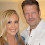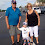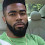## Wednesday 4 September 2013

### Linear Regression

The linear regression model is a fairly simple model, nevertheless it is used in many applications.

What follows is a fairly mathematical derivation of the linear regression parameters.

Let us denote the observations as $y[n]$ and $x[n]$ for $n=0,1,..,N-1$ for the model

$y[n] = ax[n]+b$

We assume that we can measure both $x[n]$ and $y[n]$ - the unknowns are $a$ and $b$, the gradient and intercept respectively. We can use the least squares error criterion to find estimates for $a$ and $b$, from data $x[n]$ and $y[n]$.

Let  $e[n] = y[n] - ax[n]-b$ be the error. We can represent the samples $e[n]$, $x[n]$ and $y[n]$ as length $N$ column vectors $\underline{e}$,$\underline{x}$ and $\underline{y}$ respectively.

Thus the error sum of squares (which can be regarded as a cost function $C$ to minimise over) will be

$\underline{e}^T\underline{e}=(\underline{y}-a\underline{x}-b\underline{1})^T(\underline{y}-a\underline{x}-b\underline{1}$).

where $\underline{1}$ is a column vector with all elements set to 1.

Expanding this cost function results in:-

$C=\underline{y}^T\underline{y}-2a\underline{x}^T\underline{y}-2b\underline{y}^T\underline{1}+a^2(\underline{x}^T\underline{x})+2ab(\underline{x}^T\underline{1})+b^2(\underline{1}^T\underline{1})$

Differentiating $C$ w.r.t. to $a$ and $b$:-

$\frac{\partial C}{\partial a}=2a(\underline{x}^T\underline{x})+2b(\underline{x}^T\underline{1})-2\underline{x}^T\underline{y}$

$\frac{\partial C}{\partial b}=2a(\underline{x}^T\underline{1})+2b(\underline{1}^T\underline{1})-2\underline{y}^T\underline{1}$

In order to estimate $\hat{a}$ and $\hat{b}$ (the least squares estimates of $a$ and $a$ respectively) we need to set the above two partial differential equations to 0, and solve for $a$ and $b$. We need to solve the following matrix equation

$\left[\begin{array}{cc}\underline{x}^T\underline{x}&\underline{x}^T\underline{1}\\ \underline{x}^T\underline{1}&\underline{1}^T\underline{1}\end{array}\right]$$\left[\begin{array}{c}\hat{a}\\ \hat{b}\end{array}\right] = \left[\begin{array}{c}\underline{x}^T\underline{y} \\ \underline{y}^T\underline{1} \end{array}\right] Inverting the matrix and pre multiplying both sides of the above matrix equation by the inverse results in: \left[\begin{array}{c}\hat{a} \\ \hat{b} \end{array}\right] = \frac{1}{N(\underline{x}^T\underline{x})-(\underline{x}^T\underline{1})^2}$$\left[\begin{array}{cc}\underline{1}^T\underline{1}&-\underline{x}^T\underline{1}\\ -\underline{x}^T\underline{1}&\underline{x}^T\underline{x}\end{array}\right]$$\left[\begin{array}{c}\underline{x}^T\underline{y} \\ \underline{y}^T\underline{1} \end{array}\right]$

If we revert to a more explicit notation in terms of the samples, we have

$\hat{a}=\frac{1}{N(\sum_{n=0}^{N-1}(x[n])^2)-(\sum_{n=0}^{N-1}x[n])^2}[N(\sum_{n=0}^{N-1}x[n]y[n])-(\sum_{n=0}^{N-1}y[n])(\sum_{n=0}^{N-1}x[n])]$

$\hat{b}=\frac{1}{N(\sum_{n=0}^{N-1}(x[n])^2)-(\sum_{n=0}^{N-1}x[n])^2}[(\sum_{n=0}^{N-1}(x[n])^2)(\sum_{n=0}^{N-1}y[n])-(\sum_{n=0}^{N-1}x[n])(\sum_{n=0}^{N-1}x[n]y[n])]$

where the denominator of the fraction in the above two equations is the matrix determinant.

Coefficient of Determination

Once we have calculated $\hat{a}$ and $\hat{b}$, we can see how well the linear model accounts for the data. This is achieved by finding the Coefficient of Determination ($r^2$). This is the ratio of the residual sum of squares ($rss$) to the total sum of squares ($tss$),

$\Large r^2=\frac{rss}{tss}$

and has a maximum value of 1, which is attained when the model is exactly linear. The larger $r^2$ is, the better the linear model fits the data. We can think of $r^2$ as the proportion of variation in data explained by the linear model, or the "goodness of fit" of the linear model.

The total sum of squares is given by

$tss=\sum_{n=0}^{N-1}(y[n]-\bar{y})^2$

where

$\bar{y}=\frac{1}{N}\sum_{n=0}^{N-1}y[n]$ is the mean of $y$.

The residual sum of squares ($rss$) is the total sum of squares ($tss$) minus the sum of squared errors ($sse$)

where

$sse=\sum_{n=0}^{N-1}(y[n]-\hat{a}x[n]-\hat{b})^2$

If the data perfectly fits the linear model, $sse$ will equal 0, so that $tss$ will equal $rss$ - the coefficient of determination $r^2$ will be $1$.

Summarising, we have

$\Large r^2=\frac{\sum_{n=0}^{N-1}(y[n]-\bar{y})^2-\sum_{n=0}^{N-1}(y[n]-\hat{a}x[n]-\hat{b})^2}{\sum_{n=0}^{N-1}(y[n]-\bar{y})^2}$

A worked example

Now, let us consider the following example:-

$x=[1,2,3,4]$, $y=[1.5,6,7,8]$

The matrix determinant will be $(4\times (1 + 2^2+3^2+4^2))-(1+2+3+4)^2=20$.

The least squares estimate of $a$ is

$\hat{a}=[4\times((1\times 1.5) + (2\times 6) + (3\times 7) + (4\times 8)) \\ -( (1.5+6+7+8)\times(1+2+3+4))]/20 \\ =2.05$

The least squares estimate of $b$ is

$\hat{b}=[(1+2^2+3^2+4^2)\times(1.5+6+7+8)-((1+2+3+4)\times((1\times 1.5) \\+ (2\times 6) + (3\times 7) +(4\times 8)))]/20 \\ = (675 - 665)/20 \\ =0.5$

The $tss$ is

$tss=(1.5-5.625)^2+(6-5.625)^2+(7-5.625)^2+(8-5.625)^2=24.688$

where the average of $y$ is $5.625$.

The $sse$ is

$(1.5 - (2.05\times 1) - 0.5)^2+(6 - (2.05\times 2) - 0.5)^2\\+(7 - (2.05\times 3) - 0.5)^2+(8 - (2.05\times 4) - 0.5)^2=3.675$

So that the $rss$ is $24.688-3.675=21.013$.

The coefficient of determination is

$r^2=21.013/24.688=0.8511$

This result is quite high, and is fairly indicative of a linear model.

There is a Linear Regression Calculator in this blog, which can be found here

1.All thanks to Dr Raymond for helping me to get my penis longer and bigger with his cream in just two week his cream is very active and also it work fast for everyone who have used it, the best thing about his herbal cream is that it does not have side effects and the results is permanent. Note he can also cure the below sicknesses and disease PREMATURE EJACULATIONLOW SPERM COUNTERECTILE DYSFUNCTIONHIV/AIDS CUREHERPES CUREINFECTION CUREPILESTROKEHEART FAILUREYou can email him if you need his help via: drraymondherbalcenter@gmail.com or WhatsApp him via: +2348116744524

2.I'm 40 years old female I tested genital herpes (HSV1-2) positive in 2016. I was having bad outbreaks. EXTREMELY PAINFUL. I have try different kinds of drugs and treatment by the medical doctors all to know was avail. Six months ago I was desperately online searching for a helpful remedies for genital herpes (HSV1-2) cure, which I come across some helpful remedies on how Dr OYAGU have help so many people in curing genital herpes (HSV1-2) with the help of herbal treatment because I too believe there is someone somewhere in the world who can cure herpes completely. At of the past 2 months, however, I've been following his herpes protocol Via oyaguherbalhome@gmail.com or +2348101755322 and it stopped all outbreaks completely! To my greatest surprise I was cured completely by following the protocol of his herbal medicine . Don't be discouraged by the medical doctors. There is a cure for HSV with the help of herbs and roots by a herbalist Dr call Dr OYAGU he is so kind and truthful with his herbal treatment, kindly contact him for more information Via oyaguherbalhome@gmail.com or WhatsApp DR on +2348101755322 or visit his website https://oyaguspellcaster.wixsite.com/oyaguherbalhome you will be lucky as I am today

3.God is Good! I promised God that I would share my testimony on this blog. I had all the signs of STD Virus but I was not too sure as to which one. I did a lot of online research and scared myself straight for a whole week before going to see the nurse. She took one look at my genital part and first said that it could just be the anatomy of my body, then she said it looked like genital warts and that I may have herpes. I was devastated. She gave me some medicine for the herpes and some cream for the warts. I was also tested for every single STD including herpes. I went home and cried searching the web for all sorts of cures for herpes and awaiting my results. I saw a post whereby Dr. Oyagu cured Herpes and other diseases, I copied his contacts out and added him on whats app via (+2348101755322). The next day my test result was ready and i confirmed Herpes positive. I told Dr.Oyagu about my health problems and he assured me of cure. He prepared his herbal medicine and sent it to me. I took it for 14 days (2 weeks). Before the completion of the 14 days in which I completed the dose, the Blisters and Warts that were on my body was cleared. I went back for check-up and I was told I'm free from the virus. Dr. Oyagu cures all types of diseases and viruses with the help of his herbal medicine. You can reach Dr. Oyagu via his email address on (oyahuherbalhome@gmail.com) or WhatsApp him on (+2348101755322) Visit His website on https://oyaguspellcaster.wixsite.com/oyaguherbalhome

4.I got diagnose of herpes virus last year, and i was taking some drugs prescribed for me by my family doc the drugs could not work and the herpes in my system was very terrifying that i was so depressed the good news is that i never gave up in searching for natural cure for herpes virus ,cause i believe so much in herbal medication.. One faithful morning i read a comment from a lady called Destiny Hudson on how she was cured with natural herbs made by DR.OYAGU from somewhere is West Africa, i immediately copied out his contact email via oyaguherbalhome@gmail.com and explain all my herpes problem to him via his email. the big truth is that it took just two week for his herbal medication to cure me completely without side affect. Till date am herpes virus free and all thanks to Dr OYAGU for his good deeds for me, Once again am very happy to share this great testimony of DR OYAGU cure hurry up now and contact him via his email address (oyaguherbalhome@gmail.com Call & WhatsApp him on (+2348101755322 ) and see what he can do